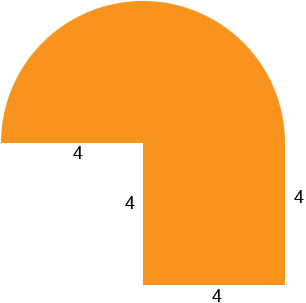SEARCH HOMEMath Central Quandaries & QueriesQuestion from Kassandra, a student: I am trying to find out the perimeter of a semicircle. I do what the book says but, I always get it wrong. I usually don't have problems with math until now. There is half a circle. It shows that the radius of the circle is 4 units. And then below the half circle there is a square below it. The angles are right angles and the width is also 4 inches. I get close to the answer but, am usually off. Please help.Hi Kassandra,

Is this the diagram you are describing?The circumference (perimeter) of a circle is 2 π r where r is the radius of the circle and π is approximately 3.1416. Thus the circumference of half a circle is π r units which for your circle is 4 π units. The remaining perimeter of the figure is made up of 4 line segments, each of length 4 units. Thus the perimeter of this figure is

4 π + 4 × 4 units.

I hope this helps,
PennyMath Central is supported by the University of Regina and The Pacific Institute for the Mathematical Sciences.